# High School Algebra: Graphing and Factoring Quadratic Equations Chapter Exam

Exam Instructions:

Choose your answers to the questions and click 'Next' to see the next set of questions. You can skip questions if you would like and come back to them later with the yellow "Go To First Skipped Question" button. When you have completed the practice exam, a green submit button will appear. Click it to see your results. Good luck!

### Page 2

#### Question 6 6. If the equation below told you a ball was 'h' feet in the air after 't' seconds, what would be the first step to finding out when it hit the ground?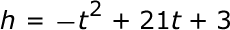#### Question 8 8. What kind of graph is shown?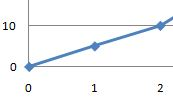### Page 3

#### Question 12 12. Rewrite the following equation in vertex form.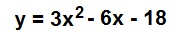#### Question 14 14. Expressing the equation below in the vertex form will give a value of 'h' = _____.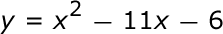### Page 4

#### Question 17 17. Factor and solve.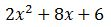### Page 5

#### Question 21 21. Find the value for c that must be added to both sides, and rewrite the equation in the vertex form.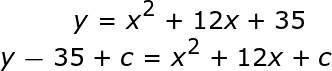#### Question 22 22. Factor.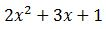#### Question 23 23. In order to complete the square on the following equation, what value would you add to both sides?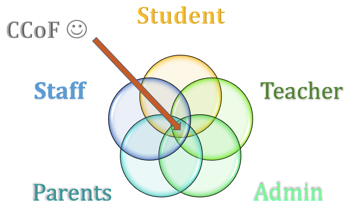#### Question 25 25. Find the solution to the equation below.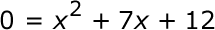### Page 6

#### Question 27 27. What kind of graph is shown?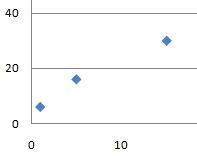#### Question 29 29. When completing the square for the quadratic equation shown below, how is c calculated?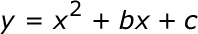#### Question 30 30. Factor.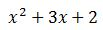#### High School Algebra: Graphing and Factoring Quadratic Equations Chapter Exam Instructions

Choose your answers to the questions and click 'Next' to see the next set of questions. You can skip questions if you would like and come back to them later with the yellow "Go To First Skipped Question" button. When you have completed the practice exam, a green submit button will appear. Click it to see your results. Good luck!

Support